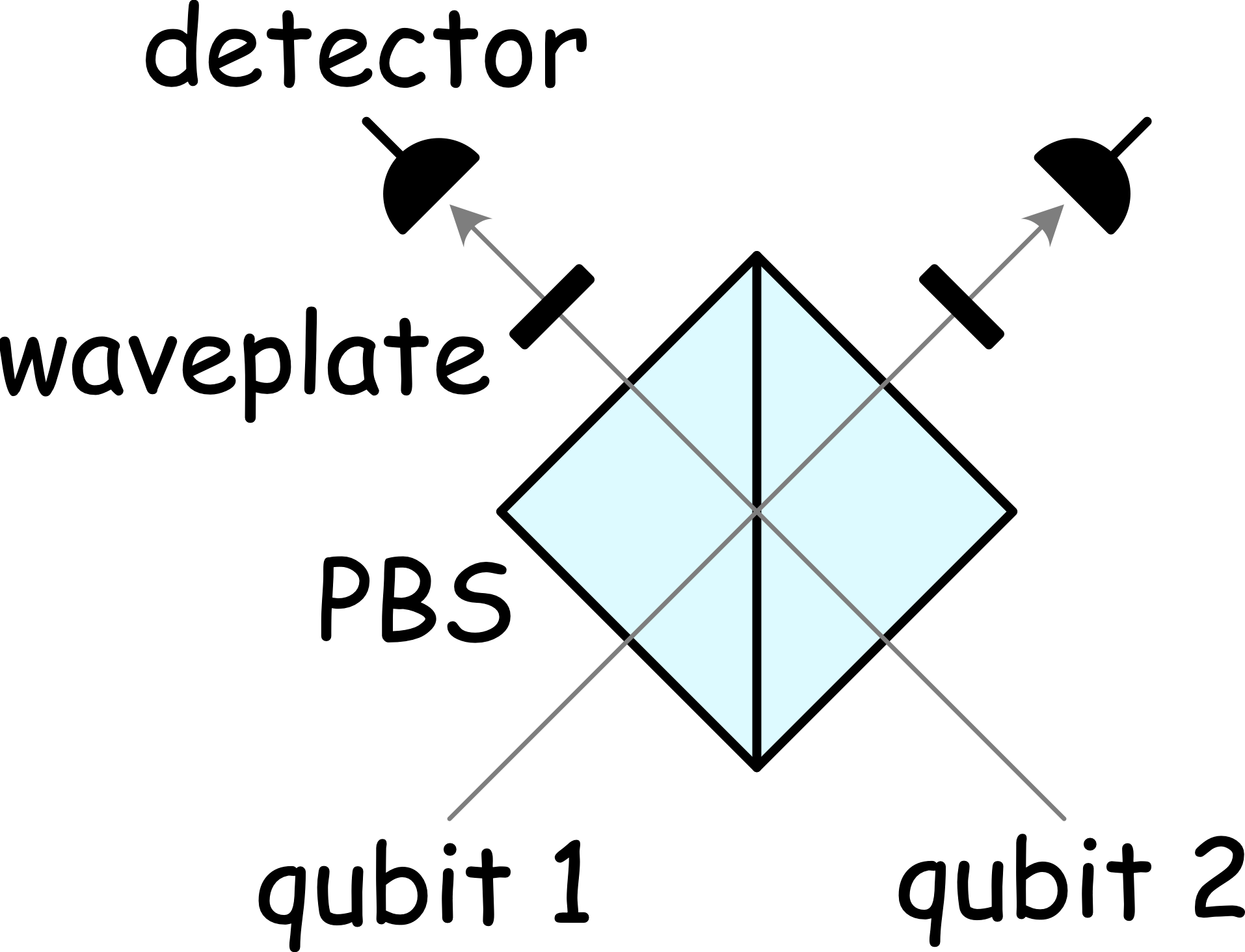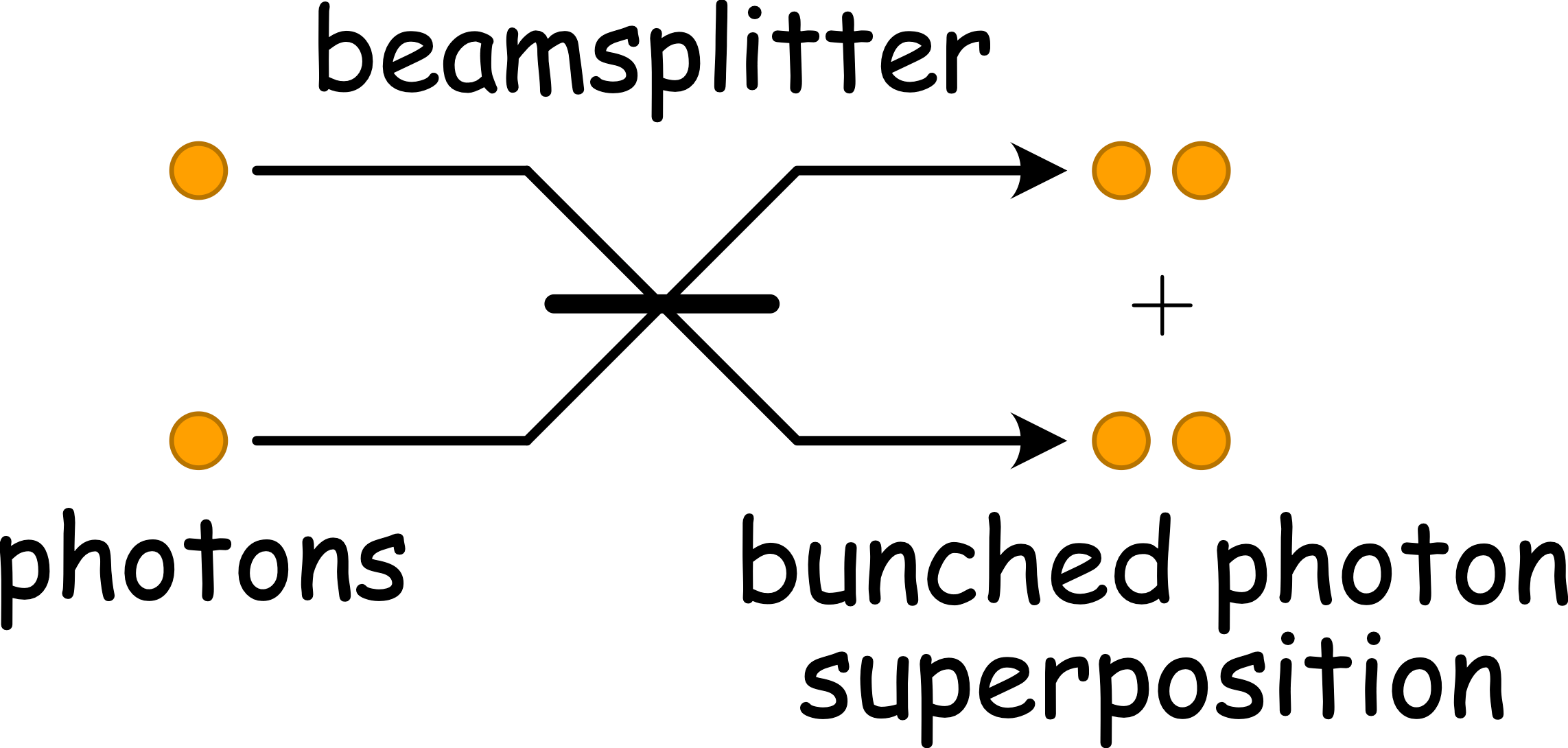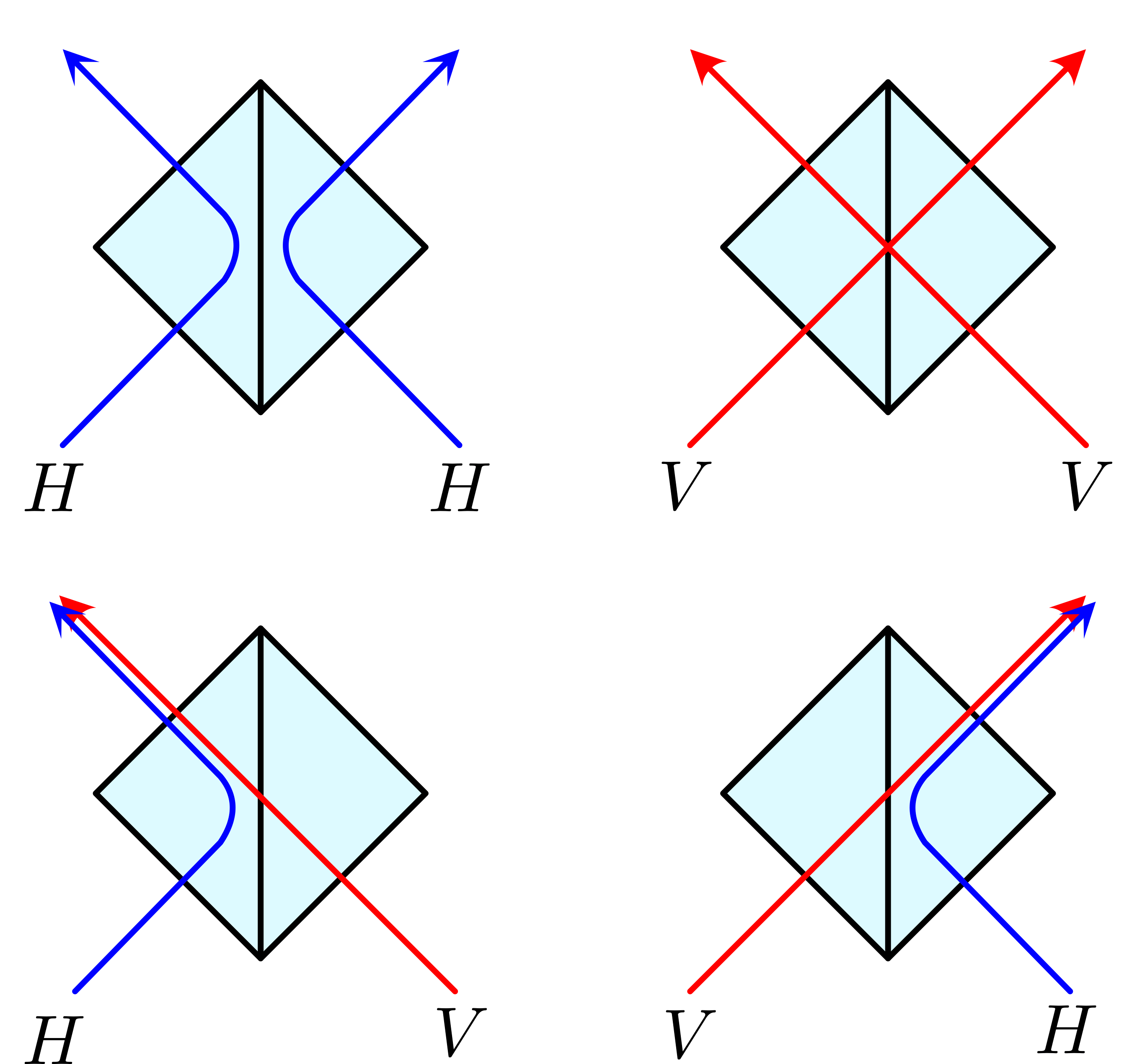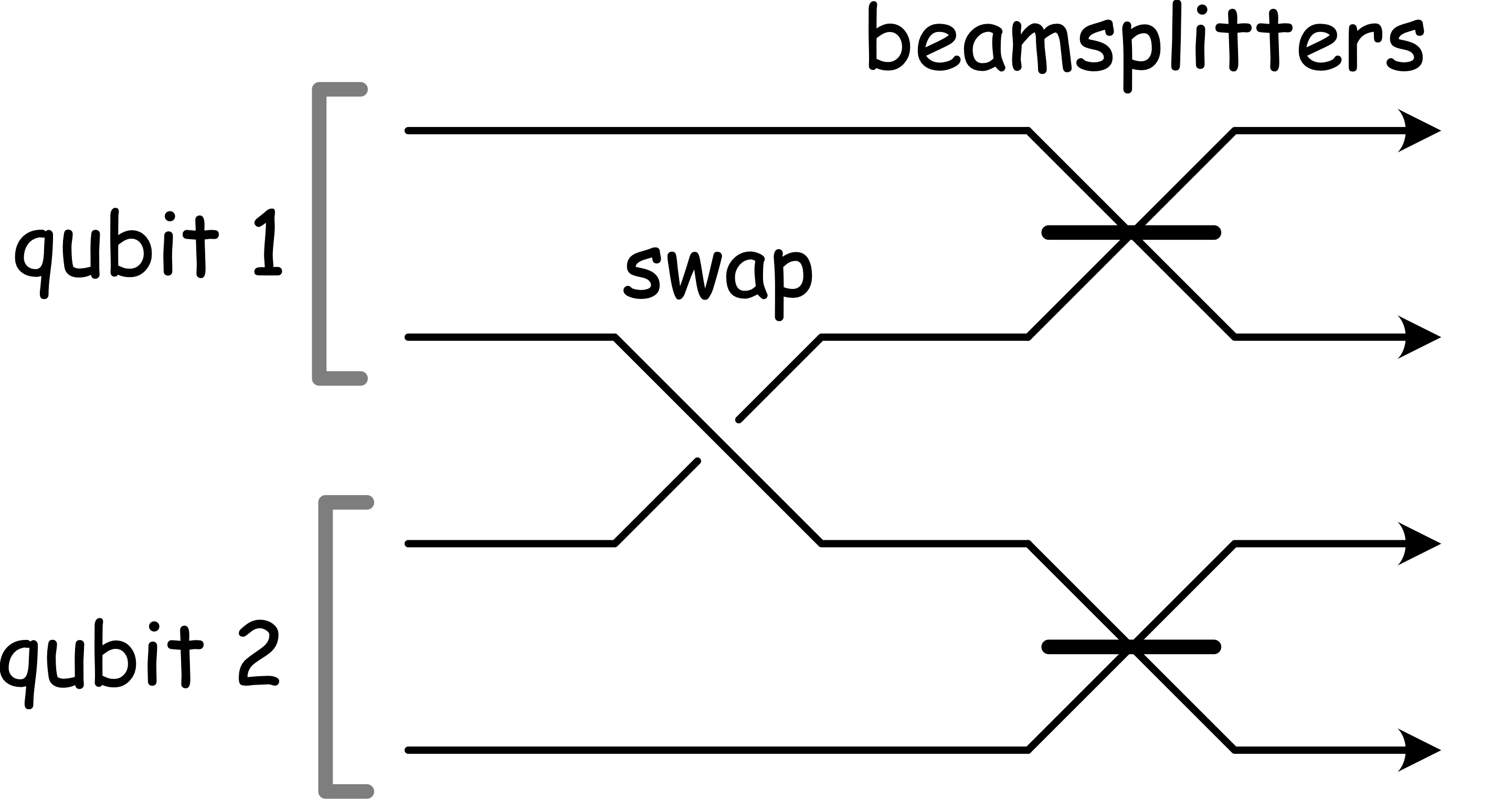# How do photonic Bell measurements work?

Entangling Bell measurements are an essential ingredient in many photonic quantum technologies. In optical quantum computing they are employed as fusion gates to create edges in graph states, while in quantum communications protocols they may be used to implement entanglement swapping in quantum repeater networks (entanglement distribution networks) for extending the range of entanglement links.

In this post I’ll describe how this very simple optical circuit works and address some of the nuances and common misconceptions surrounding it.

#### What is a Bell measurement?

A Bell measurement is a two-qubit operation that projects onto the maximally-entangled Bell basis comprising the four Bell states,

|\Phi^\pm\rangle_L = \frac{1}{\sqrt{2}}(|0,0\rangle_L \pm |1,1\rangle_L),
|\Psi^\pm\rangle_L = \frac{1}{\sqrt{2}}(|0,1\rangle_L \pm |1,0\rangle_L).

Here I’m using subscript L to denote logical qubit states. States represented without a subscript will denote Fock (or photon-number) states in an occupation number representation, where |n\rangle denotes an n-photon state.

While there are many ways in which entangling measurements can be implemented photonically, I’ll focus on by far the simplest, most well-known and widely employed implementation shown below.The partial Bell analyser for polarisation-encoded qubits, comprising a polarising beamsplitter, two waveplates implementing 45° polarisation rotations, and two polarisation-resolving photodetectors. When one photon is detected at each output (a coincidence event) we perform a partial Bell measurement.

This circuit implements a partial, destructive and non-deterministic Bell measurement. It is partial in the sense that it can only resolve two of the four Bell states. Otherwise it fails, implying non-determinism. And it is destructive in the sense that the measured qubits are destroyed by the measurement process.

The measurement projector implemented by this device is,

\hat\Pi^\pm_L =|\Phi^\pm\rangle_L\langle\Phi^\pm|_L,

a coherent projection onto one of the two even parity Bell pairs.

Bell measurements can also be implemented using CNOT gates, in which case all four Bell states can be non-destructively resolved. However, CNOT gates are notoriously difficult to construct in an optical setting, are non-deterministic, and have significant resource overheads.

#### Beamsplitters

A regular beamsplitter implements a 2\times 2 unitary transformation on the photon creation operators associated with two spatial modes, which we will denote \hat{a}^\dag_1 and \hat{a}^\dag_2,

\begin{bmatrix} \hat{a}^\dag_1 \\ \hat{a}^\dag_2\end{bmatrix} \to \begin{bmatrix} U_{1,1} & U_{1,2} \\ U_{2,1} & U_{2,2}\end{bmatrix} \begin{bmatrix} \hat{a}^\dag_1 \\ \hat{a}^\dag_2\end{bmatrix}.

Here we’re modeling evolution in the Heisenberg picture, representing state evolution via transformations on the photon creation operators acting on the vacuum state. This is the most convenient approach since all the operations we consider are represented by linear transformations of creation operators, hence the term linear optics.

For a balanced 50/50 beamsplitter we have,

U = \frac{1}{\sqrt{2}}\begin{bmatrix} 1 & 1 \\ 1 & -1\end{bmatrix},

which is recognisable as the 2\times 2 Hadamard matrix.

This is an entangling operation as it can easily be seen that the state,

|1,0\rangle = \hat{a}^\dag_1|vac\rangle,

is evolved to,

\frac{1}{\sqrt{2}}(\hat{a}^\dag_1 + \hat{a}^\dag_2)|vac\rangle = \frac{1}{\sqrt{2}}(|1,0\rangle + |0,1\rangle),

a Bell state encoded as a superposition of a single particle across two orthogonal modes.A single photon incident upon a regular beamsplitter creates an entangled output state of a superposition of a single photon across two spatial modes.

#### Polarisation rotations

A polarisation rotation, usually implemented using waveplates in experiments, implements exactly the same transformation in the polarisation degree of freedom,

\begin{bmatrix} \hat{h}^\dag \\ \hat{v}^\dag \end{bmatrix} \to\begin{bmatrix} U_{1,1} & U_{1,2} \\ U_{2,1} & U_{2,2}\end{bmatrix} \begin{bmatrix} \hat{h}^\dag \\ \hat{v}^\dag \end{bmatrix},

where \hat{h}^\dag and \hat{v}^\dag denote creation operators associated with horizontal and vertical polarisation.

Hence an input state,

\hat{h}^\dag|vac\rangle= |1\rangle_H|0\rangle_V,

is evolved by the Hadamard matrix to,

\frac{1}{\sqrt{2}}(\hat{h}^\dag + \hat{v}^\dag)|vac\rangle = \frac{1}{\sqrt{2}}(|1\rangle_H|0\rangle_V + |0\rangle_H|1\rangle_V).

Indeed, beamsplitters and polarisation rotations are isomorphic operations, implementing identical optical transformations, differing only in which pair of modes they operate on.

#### Hong-Ou-Mandel interference

Hong-Ou-Mandel (HOM) interference is a famous interferometric experiment in which a 50/50 beamsplitter interferes two photons, one incident upon each beamsplitter input.In Hong-Ou-Mandel (HOM) interference, a balanced 50/50 beamsplitter with a single photon incident at each input mode creates an equal superposition of both photons in one mode or the other at the output, known as photon bunching. These measurement statistics are uniquely quantum. In the equivalent classical experiment where each photon has 50% probability of reaching each output we would observe anti-bunched events with 50% probability.

Using the 50/50 beamsplitter transformation, an initial state with a single photon at each input,

\hat{a}^\dag_1 \hat{a}^\dag_2 |vac\rangle = |1,1\rangle,

transforms to,

\frac{1}{2}(\hat{a}^\dag_1 + \hat{a}^\dag_2)(\hat{a}^\dag_1 - \hat{a}^\dag_2)|vac\rangle = \frac{1}{\sqrt{2}}(|2,0\rangle - |0,2\rangle),

a superposition of two photons in one spatial output or two in the other. Note there is no |1,1\rangle term, as these have cancelled via destructive interference. This phenomenon is called photon bunching, as the photons ‘bunch’ together and never appear at different outputs, a uniquely quantum effect. Contrast this with classical statistics where we would expect to see anti-bunching (one particle at each output) 50% of the time.

We can replicate the same phenomenon using polarisation encoding by commencing with a two-photon state, where one is horizontally polarised, the other vertically,

\hat{h}^\dag \hat{v}^\dag |vac\rangle = |1\rangle_H|1\rangle_V,

which transforms to,

\frac{1}{2}(\hat{h}^\dag + \hat{v}^\dag)(\hat{h}^\dag - \hat{v}^\dag)|vac\rangle = \frac{1}{\sqrt{2}}(|2\rangle_H |0\rangle_V - |0\rangle_H|2\rangle_V).

#### Polarising beamsplittersEvolution of the four polarisation-encoded basis states through a polarising beamsplitter, which reflects horizontal (H) polarisation and transmits vertical (V) polarisation. When both photons have the same polarisation (even parity) we observe anti-bunching (or coincidence) events, whereas when polarisations differ (odd parity) we observe bunching. Post-selecting upon coincidence events projects us into the even parity subspace.

A polarising beamsplitter (PBS) operates very differently than a regular beamsplitter, acting on two spatial degrees of freedom, each of which is associated with two polarisation degrees of freedom, making it a four-mode transformation. Most commonly, PBS’s completely reflect one polarisation (say H) while completely transmitting the other (V), in which case the 4\times 4 transformation is,

\begin{bmatrix} \hat{h}_1^\dag \\ \hat{h}_2^\dag \\ \hat{v}^\dag_1 \\ \hat{v}^\dag_2 \end{bmatrix} \to \begin{bmatrix} 1 & 0 & 0 & 0 \\ 0 & 1 & 0 & 0 \\ 0 & 0 & 0 & 1 \\ 0 & 0 & 1 & 0 \end{bmatrix} \begin{bmatrix} \hat{h}_1^\dag \\ \hat{h}_2^\dag \\ \hat{v}^\dag_1 \\ \hat{v}^\dag_2 \end{bmatrix}.

It can be seen that this operation simply permutes modes, leaving the \hat{h}^\dag_1 and \hat{h}^\dag_2 operators unchanged, whilst swapping the \hat{v}^\dag_1 and \hat{v}^\dag_2 operators.

Beginning with any initially separable state in the original H/V basis, this operation preserves separability and cannot introduce entanglement, nor does any interference take place. Note that while this 4\times 4 matrix corresponds to that of a CNOT gate, this is not a CNOT operation as this matrix describes a transformation on creation operators not qubits.

#### Single-photon qubits

In the field of photonic quantum computing, qubits are most commonly encoded in one of two ways: dual-rail encoding and polarisation encoding. In dual-rail encoding we encode a qubit as a single photon in superposition across two distinct spatial modes. In polarisation encoding we encode a single photon in superposition across two polarisation states.

Using these two encodings, a single logical qubit,

|\psi\rangle_L = \alpha|0\rangle_L + \beta|1\rangle_L,

can be written as,

|\psi\rangle_\mathrm{dual-rail} = \alpha|1,0\rangle + \beta|0,1\rangle,
|\psi\rangle_\mathrm{polarisation} = \alpha |1\rangle_H|0\rangle_V + \beta|0\rangle_H|1\rangle_V.

Using photonic creation operators we can equivalently express these as,

|\psi\rangle_\mathrm{dual-rail} = (\alpha \hat{a}_1^\dag + \beta \hat{a}_2^\dag)|vac\rangle,
|\psi\rangle_\mathrm{polarisation} = (\alpha \hat{h}^\dag + \beta \hat{v}^\dag)|vac\rangle.

Note that in an occupation number representation both of these can be expressed,

|\psi\rangle = \alpha |1,0\rangle + \beta|0,1\rangle,

where for dual-rail encoding the two modes are spatial modes, while for polarisation encoding they refer to the two polarisation modes.

There also exists single-rail encoding, whereby a qubit is encoded in a single mode as a superposition of 0 or 1 photons. The Bell state created previously at the output of a 50/50 beamsplitter fed with a single photon input is an example of single-rail encoding. However this type of encoding has limited utility as implementing operations on single-rail qubits is highly impractical. Since the two logical basis states have different photon-number, hence energy, single-qubit gates require coherently manipulating a superposition of different amounts of energy.

In the \{|1,0\rangle,|0,1\rangle\} occupation number basis the beamsplitter and polarisation rotation operations both implement the transformations,

\begin{bmatrix} |1,0\rangle \\ |0,1\rangle \end{bmatrix} \to \begin{bmatrix} U_{1,1} & U_{1,2} \\ U_{2,1} & U_{2,2} \end{bmatrix} \begin{bmatrix} |1,0\rangle \\ |0,1\rangle \end{bmatrix},

in their respective degrees of freedom. Defining the logical basis states of a single qubit as,

|0\rangle_L \cong |1,0\rangle,
|1\rangle_L \cong |0,1\rangle,

we see that the beamsplitter and polarisation rotation operations implement 2\times 2 single-qubit unitary transformations.

So while beamsplitters and polarisation rotations are entangling operations on two optical modes, they represent single-qubit (hence non-entangling) operations when acting on qubits defined over the single-photon symmetric subspace of two modes. We refer to this as a symmetric subspace since the qubit space is invariant under permutations of the constituent optical modes. That is, any permutation of the optical modes, of which there are two (identity or swap), leaves the basis \{|1,0\rangle,|0,1\rangle\} unchanged.

#### Partial Bell measurements

Consider two arbitrary multi-qubit systems, |\psi\rangle and |\phi\rangle. Applying a Schmidt decomposition to both systems, separating out one polarisation-encoded qubit from each, which we will subsequently perform Bell measurement on,

|\psi\rangle = \alpha_0 |\psi_0\rangle|H\rangle + \alpha_1 |\psi_1\rangle|V\rangle \\|\phi\rangle = \beta_0 |\phi_0\rangle|H\rangle + \beta_1 |\phi_1\rangle|V\rangle.

Expanding this out and expressing the isolated qubits in terms of creation operators we have,

(\alpha_0\beta_0 |\psi_0\rangle |\phi_0\rangle \hat{h}^\dag_1 \hat{h}^\dag_2 + \alpha_0\beta_1 |\psi_0\rangle |\phi_1\rangle \hat{h}^\dag_1 \hat{v}^\dag_2 \\+ \alpha_1\beta_0 |\psi_1\rangle |\phi_0\rangle \hat{v}^\dag_1 \hat{h}^\dag_2 + \alpha_1\beta_1 |\psi_1\rangle |\phi_1\rangle \hat{v}^\dag_1 \hat{v}^\dag_2)|vac\rangle.

Evolving this through the PBS we obtain,

(\alpha_0\beta_0 |\psi_0\rangle |\phi_0\rangle \hat{h}^\dag_1 \hat{h}^\dag_2 + \alpha_0\beta_1 |\psi_0\rangle |\phi_1\rangle \hat{h}^\dag_1 \hat{v}^\dag_1 \\+ \alpha_1\beta_0 |\psi_1\rangle |\phi_0\rangle \hat{v}^\dag_2 \hat{h}^\dag_2 + \alpha_1\beta_1 |\psi_1\rangle |\phi_1\rangle \hat{v}^\dag_2 \hat{v}^\dag_1)|vac\rangle.

Considering only the coincidence terms we post-select upon where each spatial output has exactly one photon this reduces to,

(\alpha_0\beta_0 |\psi_0\rangle |\phi_0\rangle \hat{h}^\dag_1 \hat{h}^\dag_2 + \alpha_1\beta_1 |\psi_1\rangle |\phi_1\rangle \hat{v}^\dag_2 \hat{v}^\dag_1)|vac\rangle.

From here if we measure the two qubits in the H/V polarisation basis, we will collapse onto either,

\alpha_0\beta_0 |\psi_0\rangle |\phi_0\rangle,

or

\alpha_1\beta_1 |\psi_1\rangle |\phi_1\rangle,

depending on whether we measure H/H or V/V.

However, what we really want is a coherent projection onto both of these terms. If instead of measuring in the H/V basis we measure in the diagonal (|\pm\rangle_L=(|0\rangle_L \pm|1\rangle_L)/\sqrt{2}) basis we achieve this. The polarisation rotations prior to the photodetectors switch us into the diagonal basis. In qubit space, the balanced 50/50 beamsplitter transformation corresponds to a Hadamard gate, as does a 45° polarisation rotation, which effectively transforms the subsequent measurement from the computational \hat{Z} basis to the diagonal \hat{X} basis.

Applying the polarisation rotation we obtain,

\frac{1}{2}[\alpha_0\beta_0 |\psi_0\rangle |\phi_0\rangle (\hat{h}^\dag_1 + \hat{v}^\dag_1) (\hat{h}^\dag_2 + \hat{v}^\dag_2) \\+ \alpha_1\beta_1 |\psi_1\rangle |\phi_1\rangle (\hat{h}^\dag_1 - \hat{v}^\dag_1) (\hat{h}^\dag_2- \hat{v}^\dag_2)]|vac\rangle.

Expanding and regrouping this expression according to the different possible measurement outcomes we can write this as,

\frac{1}{2}[(\alpha_0\beta_0 |\psi_0\rangle |\phi_0\rangle + \alpha_1\beta_1 |\psi_1\rangle |\phi_1\rangle) \hat{h}^\dag_1 \hat{h}^\dag_2 \\+ (\alpha_0\beta_0 |\psi_0\rangle |\phi_0\rangle + \alpha_1\beta_1 |\psi_1\rangle |\phi_1\rangle) \hat{v}^\dag_1 \hat{v}^\dag_2 \\+ (\alpha_0\beta_0 |\psi_0\rangle |\phi_0\rangle - \alpha_1\beta_1 |\psi_1\rangle |\phi_1\rangle) \hat{h}^\dag_1 \hat{v}^\dag_2 \\+ (\alpha_0\beta_0 |\psi_0\rangle |\phi_0\rangle - \alpha_1\beta_1 |\psi_1\rangle |\phi_1\rangle) \hat{v}^\dag_1 \hat{h}^\dag_2]|vac\rangle.

Therefore, upon measuring either H/H or V/V we obtain,

\alpha_0\beta_0 |\psi_0\rangle |\phi_0\rangle + \alpha_1\beta_1 |\psi_1\rangle |\phi_1\rangle,

whereas if we measure H/V or V/H we obtain,

\alpha_0\beta_0 |\psi_0\rangle |\phi_0\rangle - \alpha_1\beta_1 |\psi_1\rangle |\phi_1\rangle,

which are the expected outcomes upon applying the,

\hat\Pi^\pm_L = |\Phi^\pm\rangle_L\langle\Phi^\pm|_L,

projectors.

What happens if rather than measuring a coincidence event we measure both photons at one output? Referring to the previous figure we see that if the input state was \hat{h}^\dag_1\hat{v}_2^\dag|vac\rangle both photons exit the top-left output, while if the input state was \hat{v}^\dag_1\hat{h}_2^\dag|vac\rangle both photons exit the top-right output. This means that if we measure two photons at one output we know exactly what the polarisation of both inputs was. Therefore, when the device fails to project onto the even-parity subspace it performs a computational basis (\hat{Z}) measurement on both qubits.

#### Where does the entanglement come from?

The above calculation is completely legitimate, but it isn’t clear at all where the entanglement comes from in our entangling measurement. The PBS is a non-entangling operation, and both our inputs and the post-selected outputs are in the qubit basis, whereby polarisation rotations implement single-qubit operations. It sounds like everything involved is non-entangling?

The resolution to the paradox is found in the terms we post-selected away. The non-coincidence terms that we eliminated were of the form \hat{h}^\dag\hat{v}^\dag, one such term associated with each of the PBS outputs, which subsequently undergo polarisation rotation. These two-photon terms are not confined to qubit space and undergo HOM interference, creating highly entangled two-photon terms of the form \hat{h}^{\dag^2}-\hat{v}^{\dag^2}.Bell measurement circuit for dual-rail encoded qubits. The mode-swapping operation in dual-rail encoding corresponds to a polarising beamsplitter in polarisation encoding, while the beamsplitters correspond to polarisation rotations.

So while our input states can be considered polarisation encoded qubits and the overall transformation implemented by the device is a two-qubit entangling gate, internally our states are not confined to qubit space and the polarisation rotations prior to the detectors cannot be strictly considered as single-qubit gates. Rather, they are highly entangling multi-photon operations on two optical modes.

Entanglement is always defined relative to a basis and a state which is entangled in one basis needn’t be entangled in another. The most obvious example is that a Bell state is entangled in the qubit basis but not entangled in the Bell basis and vice-versa. Here we’ve defined a qubit space as the single-photon subspace of a two-mode Fock space, where entangling operations in the latter define local operations in the former.

It is correct to say that our partial Bell analyser relies on Hong-Ou-Mandel interference. But it doesn’t take place in the polarising beamsplitter, it takes place within the waveplates.

#### Polarisation-resolving photodetectors

In our optical circuit we required polarisation-resolving photodetectors. In practise, photodetectors available to us in the laboratory don’t have the ability to do this directly – they only resolve photon-number. However, this can easily be overcome by utilising an additional PBS to spatially separate and independently detect a state’s polarisation components, as shown below.Photo-detectors typically only measure photon-number but not polarisation. However, using a polarising beamsplitter we can spatially separate and independently detect the horizontal and vertical components of a polarisation-encoded qubit, thereby implementing polarisation-resolved detection.

So our original optical circuit, when experimentally implemented, will actually comprise three PBS’s and four photodetectors, and the full circuit will look like this.

(Acknowledgement: Thank you to Felix Zilk for providing very helpful feedback on this post.)

# Response to “Pause Giant AI Experiments: An Open Letter”

I completely disagree with the open letter signed by Elon Musk and numerous other luminaries in which they advocate a moratorium on advancing AI so that time can be taken to consider the implications and risks associated with this technology.

• While the intention is well-meaning and the risks are real, the analysis is superficial and unlikely to play out as suggested.

• Although there are undeniably major risks presented by advanced AI, a moratorium is unlikely to further progress in dealing with them and more likely hinder it. Political responses to disruptive forces tend to be reactionary rather than preemptive and it is not foreseeable that during such a moratorium political and regulatory solutions will be implemented. It is naive to think that if presented with a six month window of opportunity to consider the implications of AI that politicians and regulators are going to make use of it to formulate a master plan. Societal consensus and political responses to complex emerging problems do not take place over such short timescales, and attempts to do so are likely to be poorly considered.

• Such a moratorium is necessarily voluntary as there are no mechanisms for global enforcement, meaning that only good actors will participate, tilting the balance of power in the AI sphere in favour of bad actors.

• Technological advancement is inherently disruptive and there are many instances through modern history where technology has made types of human labour redundant. However, it is very clear that embracing technology has in general driven humanity forward not backward.

• Attempting to inhibit technological advancement is largely futile and unenforceable. Adapting to embrace it is by far the best approach. Adaptation is an evolutionary process, not something that can be decided in advance. We are not in a position to make advance determinations as there are too many unknowns and the spectrum of implications is unclear.

• Obstructing technological advancement that competes against us is a form of protectionism. Recently Italy placed a ban on ChatGPT, and some other EU nations are reportedly considering the same. Doing so, rather than encouraging home-grown development of AI industries represents a major economic setback, enforces competitive disadvantage, and missed opportunity that risks future economic irrelevance. This is not to say that Italy’s privacy-related concerns have no merit. However, placing an outright ban on emerging technologies, rather than adapting to them in tandem with their development is backward thinking. The same line of reasoning could equally be used to justify banning any of the cloud services we all rely on or the internet as a whole.

• Yes, advanced AI will be highly disruptive, but also transformative, with the potential to act as a huge multiplier on productivity, which drives economic progress and human development. Wilfully delaying or missing this opportunity is economically and strategically destructive, handing power to competitors and adversaries.

• We definitely should be acting quickly in considering the ethical and broader implications of AI upon society, but placing a halt on technological progress isn’t going to expedite this process. That will happen as the implications becomes tangible, and in the meantime we’ll have only delayed progress for no reason.

• Openness and transparency are the most powerful forces against malevolent misuse. Driving things underground inhibits this, imposing opaqueness on the sector.

• Turning AI into a black market is completely foolish.

# MoodSnap now in Spanish

We’re delighted to announce that MoodSnap mood diary is now localised to the Spanish language. We’re incredibly excited that all our Spanish-speaking friends can now use MoodSnap in their native language.

Thank you to Melany Nadine Monroy Icaza for providing the translation and Christian Ronald Cresci for proof-checking.

www.moodsnap.app

# Terminally Quantum podcast series

Together with Alexandra Dickie we’re pleased to announce our new quantum podcast series Terminally Quantum, hosted at The Quantum Terminal in Sydney, Australia. Our first episode, featuring Prof Peter Turner, CEO of the Sydney Quantum Academy, is now available on Spotify and Apple Podcasts.

# MoodSnap jetzt in Deutsch

One of the goals of my free mood diary app MoodSnap is to make it accessible to as many people as possible. Today I’m pleased to announce that MoodSnap has been fully localised for the German language, with more languages in progress.

You can get MoodSnap on the Apple AppStore and find out more at the MoodSnap homepage.